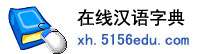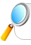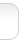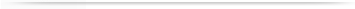汉字   按部首检索  按拼音检索雨字的男姓名 | 雨字的女姓名 | 雨开头的姓名 | 雨结尾的姓名 | 更多带雨的姓名
 雨 (男) 雨 (女) 春雨 (男) 春雨 (女) 雨薇 (女) 雨婷 (女) 雨晴 (女) 晓雨 (女) 小雨 (女) 思雨 (女) 红雨 (男) 晓雨 (男) 雨辰 (男) 雨佳 (女) 雨欣 (女) 小雨 (男) 红雨 (女) 雨晨 (女) 雨生 (男) 洪雨 (男) 诗雨 (女) 雨桐 (女) 雨田 (男) 梦雨 (女) 雨龙 (男) 雨辰 (女) 雨晨 (男) 雨涵 (女) 雨洁 (女) 雨菲 (女) 雨轩 (男) 雨萌 (女) 雨林 (男) 雨峰 (男) 雨蒙 (女) 雨竹 (女) 欣雨 (女) 庆雨 (男) 雨虹 (女) 时雨 (男) 雨彤 (女) 雨华 (男) 雨霏 (女) 雨佳 (男) 振雨 (男) 雨春 (男) 雨明 (男) 天雨 (男) 雨亭 (男) 雨青 (女) 雨松 (男) 雨馨 (女) 雨轩 (女) 雨阳 (男) 明雨 (男) 雨珊 (女) 思雨 (男) 新雨 (男) 雨红 (女) 佳雨 (女) 雨琪 (女) 秋雨 (男) 雨琴 (女) 建雨 (男) 秋雨 (女) 雨露 (女) 潇雨 (女) 雷雨 (男) 雨微 (女) 雨飞 (男) 雨萱 (女) 雨青 (男) 雨昕 (女) 风雨 (男) 雨泽 (男) 雨平 (男) 雨桐 (男) 金雨 (男) 雨来 (男) 雨航 (男) 诗雨 (男) 雨静 (女) 文雨 (男) 雨萌 (男) 雨果 (男) 雨燕 (女) 德雨 (男) 晨雨 (男) 丝雨 (女) 雨霞 (女) 雨田 (女) 雨涛 (男) 雨阳 (女) 国雨 (男) 雨潇 (男) 笑雨 (女) 雨欣 (男) 雨杉 (女) 冬雨 (男) 雨顺 (男) 雨霖 (男) 雨花 (女) 雨萍 (女) 梦雨 (男) 雨玲 (女) 兴雨 (男) 雨濠 (女) 泽雨 (男) 晨雨 (女) 兆雨 (男) 雨菡 (女) 雨梅 (女) 雨翔 (男) 雨鑫 (女) 雨晴 (男) 祥雨 (男) 雨潇 (女) 佳雨 (男) 潇雨 (男) 雨华 (女) 雨璇 (女) 志雨 (男) 文雨 (女) 雨东 (男) 心雨 (女) 雨亭 (女) 雨雷 (男) 雨鑫 (男) 雨丰 (男) 雨倩 (女) 雨琦 (女) 华雨 (男) 雨荷 (女) 雨良 (男) 雨楠 (女) 雨森 (男) 馨雨 (女) 长雨 (男) 雨舟 (男) 雨雯 (女) 光雨 (男) 雨奇 (男) 雨忠 (男) 新雨 (女) 龙雨 (男) 雨风 (男) 雨成 (男) 雨蒙 (男) 雨清 (女) 鑫雨 (男) 天雨 (女) 雨凡 (男) 雨露 (男) 雨薇 (男) 雨润 (女) 东雨 (男) 雨沁 (女) 雨锋 (男) 雨洲 (男) 雨兰 (女) 星雨 (男) 中雨 (男) 宏雨 (男) 雨芳 (女) 连雨 (男) 传雨 (男) 士雨 (男) 雨新 (男) 雨时 (男) 雨生 (女) 雨杰 (男) 雨洋 (女) 雨航 (女) 雨润 (男) 雨江 (男) 雨波 (男) 俊雨 (男) 雨宁 (女) 雨旺 (男) 雨坤 (男) 雨豪 (男) 忠雨 (男) 雨清 (男) 大雨 (男) 雨泉 (男) 雨希 (女) 雨娟 (女) 夏雨 (男) 雨珂 (女) 时雨 (女) 书雨 (男) 田雨 (男) 雨来 (女) 雨斌 (男) 敬雨 (男) 雨庭 (男) 云雨 (男) 雨声 (男) 雨朦 (女) 雨泽 (女) 善雨 (男) 仁雨 (男) 虹雨 (女) 静雨 (男) 化雨 (男) 笑雨 (男) 雨卉 (女) 雨香 (女) 昌雨 (男) 雨丽 (女) 雨洋 (男) 雨强 (男) 雨曦 (女) 雨诺 (女) 星雨 (女) 雨蓉 (女) 汉雨 (男) 雨军 (男) 雨林 (女) 雨娇 (女) 得雨 (男) 雨含 (女) 雨诗 (女) 雨平 (女) 雨辉 (男) 雨石 (男) 雨海 (男) 雨亮 (男) 雨石 (女) 孝雨 (男) 存雨 (男) 继雨 (男) 雨晗 (女) 雨宸 (女) 若雨 (女) 雨姣 (女) 雨婷 (男) 雨思 (女) 胜雨 (男) 雨根 (男) 辰雨 (男) 雨嘉 (男) 雨莹 (女) 雨杭 (男) 逢雨 (男) 雨飞 (女) 雨春 (女) 雨濛 (女) 雨浓 (女) 雨雨 (女) 雨灵 (女) 倩雨 (女) 雨川 (男) 正雨 (男) 田雨 (女) 雨楠 (男) 作雨 (男) 向雨 (男) 恒雨 (男) 清雨 (男) 雨冰 (女) 雨珍 (女) 雨溪 (女) 雨函 (女) 雨茜 (女) 雨竹 (男) 道雨 (男) 谷雨 (男) 沁雨 (女) 雨梦 (女) 雨帆 (男) 雨秋 (女) 雨男 (男) 凤雨 (男) 夭雨 (男) 雨璐 (女) 雨宏 (男) 良雨 (男) 雨农 (男) 广雨 (男) 欣雨 (男) 顺雨 (男) 雨昕 (男) 雨婕 (女) 雨琳 (女) 雨莲 (女) 吉雨 (男) 雨瞳 (女) 雨菊 (女) 巧雨 (女) 雨文 (男) 雨瑶 (女) 雨浓 (男) 雨滋 (男) 绍雨 (男) 雨广 (男) 雨涵 (男) 朝雨 (男) 青雨 (男) 斯雨 (女) 雨星 (男) 雨艳 (女) 雨君 (女) 飞雨 (男) 冬雨 (女) 泽雨 (女) 朋雨 (男) 守雨 (男) 雨山 (男) 雨茹 (女) 雨时 (女) 雨奇 (女) 雨丝 (女) 雨芬 (女) 维雨 (男) 桐雨 (女) 雨芹 (女) 永雨 (男) 其雨 (男) 雨庆 (男) 雨嘉 (女) 雨寒 (女) 雨光 (男) 雨凝 (女) 雨蔚 (女) 霄雨 (女) 树雨 (女) 学雨 (男) 甜雨 (女) 雨安 (女) 雨红 (男) 雨凌 (女) 雨杉 (男) 成雨 (男) 雨雪 (男) 昕雨 (女) 雨人 (男) 雨衡 (男) 洪雨 (女) 雨纯 (女) 雨朋 (男) 培雨 (男) 秀雨 (男) 雨心 (女) 若雨 (男) 雨钢 (男) 荣雨 (男) 万雨 (男) 雨霁 (女) 雨卓 (女) 雨乔 (女) 化雨 (女) 雨琦 (男) 雨虹 (男) 雨水 (男) 雨凡 (女) 军雨 (男) 夏雨 (女) 金雨 (女) 冰雨 (男) 雨彬 (男) 谷雨 (女) 彦雨 (男) 肖雨 (女) 静雨 (女) 雨声 (女) 雨鹏 (男) 雨叶 (女) 宗雨 (男) 雨凤 (女) 成雨 (女) 雨谦 (女) 沁雨 (男) 子雨 (男) 先雨 (男) 雨和 (男) 雨雨 (男) 海雨 (男) 雨果 (女) 建雨 (女) 嘉雨 (男) 保雨 (男) 尚雨 (男) 雨岑 (女) 雨伟 (男) 会雨 (男) 雨涛 (女) 瑞雨 (男) 凡雨 (男) 雨兴 (男) 雨甜 (女) 雨英 (女) 雨禾 (女) 雨杨 (女) 雨兵 (男) 雨乐 (女) 筱雨 (女) 舒雨 (女) 冰雨 (女) 雨民 (男) 章雨 (男) 雨村 (男) 雨寒 (男) 桂雨 (男) 振雨 (女) 立雨 (男) 雨夏 (男) 雨菲 (男) 少雨 (男) 寒雨 (男) 雨嫣 (女) 舒雨 (男) 雨星 (女) 翔雨 (男) 雨枫 (男) 世雨 (男) 雨冬 (男) 清雨 (女) 雨舟 (女) 雨云 (女) 嘉雨 (女) 江雨 (男) 雨潼 (女) 雨文 (女) 令雨 (男) 芯雨 (女) 泉雨 (男) 雨童 (男) 雨鸥 (男) 雨超 (男) 丙雨 (男) 雨勤 (男) 雨曦 (男) 正雨 (女) 雨云 (男) 雨悦 (女) 鸿雨 (男) 雨琼 (女) 雨锡 (男) 夭雨 (女) 平雨 (男) 雨珠 (女) 雨墨 (男) 雨芯 (女) 元雨 (男) 方雨 (男) 雨霏 (男) 丰雨 (男) 雨宝 (男) 雨池 (男) 听雨 (女) 一雨 (男) 弘雨 (男) 燕雨 (女) 扬雨 (男) 雨洲 (女) 雨点 (女) 雨骁 (男) 雨贵 (男) 雨蔓 (女) 筱雨 (男) 雨婧 (女) 广雨 (女) 曦雨 (女) 雨默 (男) 昭雨 (男) 华雨 (女) 虹雨 (男) 雨苗 (男) 雨昌 (男) 淑雨 (女) 轩雨 (女) 雨涌 (男) 雨宸 (男) 雨潭 (男) 鹏雨 (男) 雨蕾 (女) 雨伦 (女) 雨馨 (男) 雨昊 (男) 明雨 (女) 雨贤 (女) 雨柔 (女) 雨尧 (男) 震雨 (男) 雨樵 (男) 雨安 (男) 雨迪 (女) 雨虎 (男) 雨康 (男) 雨作 (男) 贵雨 (男) 怀雨 (男) 雪雨 (男) 雨菱 (女) 双雨 (女) 雨可 (女) 雨妍 (女) 雨初 (男) 雨利 (男) 雨童 (女) 方雨 (女) 霄雨 (男) 雨冰 (男) 雨菅 (女) 爱雨 (女) 开雨 (男) 雨官 (男) 增雨 (男) 雨晖 (女) 雨祥 (男) 铭雨 (男) 耿雨 (男) 雨新 (女) 雨钊 (男) 靖雨 (女) 雨然 (女) 雨廷 (男) 洁雨 (女) 雨酥 (女) 雨冉 (女) 更雨 (男) 雨天 (男) 雨君 (男) 雨风 (女) 家雨 (男) 兆雨 (女) 启雨 (男) 歆雨 (女) 雨恒 (男) 瑞雨 (女) 雨西 (女) 雨宁 (男) 景雨 (男) 夕雨 (女) 雨非 (男) 雨桦 (女) 恩雨 (男) 殿雨 (男) 艳雨 (女) 沛雨 (男) 雨哲 (男) 喜雨 (女) 雨秀 (女) 雨笛 (女) 雨栋 (男) 知雨 (女) 甘雨 (男) 雨彤 (男) 中雨 (女) 雨法 (男) 雨喜 (男) 雨城 (男) 雨昆 (男) 雨龙 (女) 雨壮 (男) 净雨 (女) 咏雨 (男) 雨哲 (女) 晶雨 (女) 雨达 (男) 宛雨 (女) 雨洁 (男) 召雨 (男) 好雨 (男) 桐雨 (男) 龙雨 (女) 雨徼 (男) 雨玫 (女) 旭雨 (男) 雨程 (男) 沛雨 (女) 雨樱 (女) 雨晗 (男) 孝雨 (女) 桂雨 (女) 雨南 (女) 来雨 (男) 雨淋 (女) 雨媛 (女) 雨贝 (女) 雨诚 (男) 雨立 (男) 雨乐 (男) 雷雨 (女) 雨姝 (女) 雨歆 (女) 群雨 (男) 雨喜 (女) 雨友 (男) 雨笑 (女) 兰雨 (男) 雨升 (男) 雨央 (女) 雨激 (女) 忠雨 (女) 雨娇 (男) 雨姗 (女) 佰雨 (男) 雨健 (男) 私雨 (女) 志雨 (女) 雨歌 (女) 雨巍 (男) 雨毅 (男) 云雨 (女) 雨腾 (男) 灏雨 (男) 雨申 (男) 雨巧 (女) 雨晰 (女) 雨潞 (女) 雨苓 (女) 吟雨 (女) 洁雨 (男) 京雨 (男) 宏雨 (女) 雨烟 (女) 雨俊 (男) 斯雨 (男) 丹雨 (女) 歆雨 (男) 雨失 (男) 祖雨 (男) 贺雨 (男) 雨汛 (男) 非雨 (女) 风雨 (女) 雨茁 (男) 雨捷 (男) 沐雨 (男) 雨朦 (男) 显雨 (男) 昶雨 (女) 雨震 (男) 雨婵 (女) 雨麦 (男) 雨晓 (女) 雨银 (男) 树雨 (男) 雨后 (男) 雨垄 (女) 默雨 (女) 雨杨 (男) 雨淇 (女) 雨甜 (男) 添雨 (女) 铮雨 (女) 雨昂 (男) 雨兮 (女) 雨晃 (男) 雨男 (女) 智雨 (男) 好雨 (女) 雨盛 (男) 雨烨 (女) 雨谡 (男) 雨翰 (男) 雨均 (女) 楚雨 (男) 书雨 (女) 为雨 (男) 雨晖 (男) 雨檬 (女) 雨淋 (男) 桑雨 (女) 雨宏 (女) 雨楫 (男) 雨如 (女) 雨濛 (男) 雨燃 (男) 雨胜 (男) 俊雨 (女) 雨巍 (女) 坤雨 (男) 伏雨 (男) 雨菡 (男) 雨菁 (女) 雨赋 (男) 雨晟 (男) 苗雨 (男) 雨川 (女) 雨杭 (女) 雨芊 (男) 雨谖 (女) 和雨 (男) 雨靖 (男) 双雨 (男) 雨建 (男) 韩雨 (女) 雨桉 (女) 雨寰 (女) 高雨 (男) 本雨 (男) 雨洪 (女) 雨波 (女) 雨希 (男) 常雨 (男) 登雨 (男) 雨垄 (男) 雨勳 (男) 雨铭 (男) 晗雨 (女) 辰雨 (女) 雨筱 (女) 慧雨 (女) 雨思 (男) 雨舒 (女) 恩雨 (女) 雨传 (男) 雨颖 (女) 剑雨 (男) 雨伦 (男) 梨雨 (女) 雨侬 (女) 秀雨 (女) 雨彦 (女) 雨正 (男) 雨峰 (女) 凤雨 (女) 之雨 (男) 雨卓 (男) 雨楼 (男) 鑫雨 (女) 雨恬 (男) 雨刚 (男) 君雨 (男) 雨诗 (男) 雨人 (女) 刚雨 (男) 雨昀 (女) 雨晔 (男) 雨雷 (女) 奎雨 (男) 雨柯 (女) 雨非 (女) 婧雨 (女) 嫣雨 (女) 雨若 (男) 美雨 (女) 山雨 (男) 雨善 (男) 雨琪 (男) 阳雨 (女) 雨挺 (男) 宣雨 (男) 湘雨 (男) 雨恬 (女) 君雨 (女) 雨泓 (女) 巨雨 (男) 雨妹 (女) 雨然 (男) 从雨 (男) 布雨 (男) 雨敏 (女) 靖雨 (男) 孟雨 (女) 雨羚 (女) 雨枷 (女) 飘雨 (女) 传雨 (女) 雨含 (男) 雨泓 (男) 占雨 (男) 牧雨 (女) 蒙雨 (女) 雨白 (男) 露雨 (女) 雨恩 (男) 雨谷 (男) 班雨 (男) 雨隆 (男) 雨雪 (女) 雨昱 (男) 雨擎 (女) 利雨 (男) 雨雁 (女) 雨霖 (女) 伟雨 (男) 雨帆 (女) 厚雨 (男) 雨才 (男) 雨昆 (女) 雨丹 (女) 雨谦 (男) 辛雨 (女) 雨旋 (女) 雨聪 (男) 雨范 (女) 雨福 (男) 跃雨 (男) 雨萧 (女) 雨志 (男) 治雨 (男) 同雨 (男) 雨成 (女) 雨棋 (男) 辛雨 (男) 纪雨 (男) 晴雨 (女) 雨影 (女) 宵雨 (女) 雨德 (男) 琛雨 (女) 雨大 (男) 雨婪 (女) 雨汀 (女) 陈雨 (女) 雨燕 (男) 廷雨 (男) 雨坪 (男) 雨苗 (女) 纯雨 (男) 友雨 (男) 雨沛 (男) 克雨 (男) 雨凯 (男) 雨栖 (女) 雨俏 (女) 银雨 (男) 宝雨 (男) 雨臣 (男) 雨堃 (男) 灵雨 (女) 雨修 (男) 圣雨 (男) 菡雨 (女) 旱雨 (男) 雨淳 (女) 雨笋 (男) 含雨 (男) 茂雨 (男) 雨晶 (女) 帮雨 (男) 雨临 (男) 镇雨 (男) 雨祺 (男) 施雨 (女) 雨静 (男) 贵雨 (女) 雨芄 (女) 多雨 (男) 沐雨 (女) 锦雨 (男) 雨慧 (男) 雨停 (男) 霓雨 (男) 雨练 (男) 雨碧 (女) 雨寰 (男) 滋雨 (男) 雨吟 (男) 致雨 (男) 雨象 (男) 落雨 (女) 年雨 (男) 雨可 (男) 婉雨 (男) 雨芄 (男) 坤雨 (女) 示雨 (女) 航雨 (男) 雨夜 (女) 雨遥 (男) 雨付 (男) 雨娜 (女) 学雨 (女) 雨淑 (女) 雨霈 (女) 雨舒 (男) 雨熙 (女) 雨彻 (男) 绪雨 (女) 锡雨 (男) 乐雨 (男) 雨沛 (女) 战雨 (男) 雨振 (男) 远雨 (男) 林雨 (女) 雨滢 (男) 雨剑 (男) 雨雕 (男) 鉴雨 (男) 显雨 (女) 径雨 (男) 雨嵩 (男) 子雨 (女) 雨策 (男) 雨路 (男) 雨皙 (男) 雨淳 (男) 雨鸿 (女) 雨得 (男) 修雨 (女) 雨芝 (女) 陆雨 (男) 奇雨 (女) 雨寿 (男) 霁雨 (女) 添雨 (男) 雨舲 (男) 雨富 (男) 雨橙 (男) 雨湘 (女) 北雨 (男) 姿雨 (男) 淑雨 (男) 驰雨 (男) 雨尘 (女) 雨珩 (男) 润雨 (女) 雨量 (男) 润雨 (男) 雨杰 (女) 雨滋 (女) 赤雨 (男) 雨奔 (男) 梅雨 (男) 雨默 (女) 江雨 (女) 雨禅 (女) 如雨 (男) 雨濂 (女) 雨寅 (男) 钲雨 (男) 雨沁 (男) 雨伶 (男) 啊雨 (男) 甘雨 (女) 雨少 (男) 雨棠 (男) 稼雨 (男) 舟雨 (女) 雨舰 (男) 雨臻 (男) 福雨 (女) 雨慷 (男) 雨霆 (男) 名雨 (男) 古雨 (男) 顶雨 (男) 警雨 (女) 雨霜 (男) 宁雨 (男) 效雨 (男) 杏雨 (女) 雨桦 (男) 雨爽 (女) 雨丹 (男) 亦雨 (女) 雨书 (女) 聪雨 (男) 雨芸 (女) 雨佶 (女) 雨园 (女) 雨棋 (女) 雨岑 (男) 骆雨 (女) 雨屏 (女) 雨珂 (男) 雨群 (男) 雨子 (男) 池雨 (男) 微雨 (女) 雨美 (女) 逢雨 (女) 夜雨 (女) 雨汾 (女) 雨端 (女) 晴雨 (男) 涵雨 (女) 美雨 (男) 石雨 (男) 雨娉 (女) 雨亮 (女) 昆雨 (男) 雨舸 (女) 自雨 (男) 雨蕊 (女) 雨录 (男) 象雨 (男) 言雨 (女) 湘雨 (女) 佃雨 (男) 雨兵 (女) 行雨 (男) 弨雨 (男) 雨播 (男) 雨适 (男) 雨茂 (男) 雨啸 (女) 孙雨 (男) 陈雨 (男) 雨玖 (女) 雨莉 (女) 佩雨 (女) 彤雨 (男) 雨山 (女) 雨肖 (男) 雨澄 (女) 丝雨 (男) 尧雨 (男) 雨齐 (女) 伯雨 (男) 源雨 (女) 雨培 (男) 雨壕 (男) 雨白 (女) 雨方 (男) 雨镖 (男) 雨瑜 (女) 雨年 (男) 雨多 (男) 雨妡 (女) 辉雨 (女) 鸣雨 (女) 雨骋 (男) 雨俊 (女) 禧雨 (女) 耕雨 (男) 雨校 (女) 雨环 (男) 詹雨 (男) 雨竺 (女) 孟雨 (男) 亮雨 (男) 雨驰 (男) 雨俭 (男) 雨艳 (男) 雨荣 (女) 勤雨 (女) 楚雨 (女) 雨前 (女) 茗雨 (女) 素雨 (男) 雨溕 (女) 沫雨 (男) 业雨 (男) 雨墨 (女) 可雨 (男) 澎雨 (女) 朝雨 (女) 马雨 (男) 步雨 (男) 泓雨 (男) 雨深 (男) 雨勇 (女) 昶雨 (男) 苏雨 (男) 念雨 (女) 宜雨 (男) 雨芙 (女) 雨啸 (男) 歌雨 (男) 宗雨 (女) 翃雨 (男) 夕雨 (男) 雨禄 (女) 雨倩 (男) 铮雨 (男) 婕雨 (女) 获雨 (女) 送雨 (女) 雨裙 (女) 换雨 (女) 雨炜 (女) 窗雨 (男) 雨怡 (男) 雨旷 (男) 雨珠 (男) 雨渤 (女) 雨肖 (女) 竣雨 (男) 宇雨 (女) 雨桓 (女) 喜雨 (男) 扬雨 (女) 庆雨 (女) 房雨 (男) 雨芮 (男) 衡雨 (男) 尔雨 (女) 仟雨 (女) 雨军 (女) 少雨 (女) 皆雨 (男) 雨捷 (女) 雨晏 (女) 修雨 (男) 洋雨 (男) 程雨 (女) 雨晓 (男) 品雨 (男) 盈雨 (女) 忻雨 (男) 雨崇 (男) 舜雨 (女) 雨吉 (男) 雨劲 (男) 雨之 (男) 晋雨 (男) 皆雨 (女) 雨秋 (男) 雨土 (女) 雨蚊 (男) 荣雨 (女) 雨贤 (男) 雨璟 (男) 雨鸣 (女) 玉雨 (女) 雨枫 (女) 杰雨 (男) 心雨 (男) 浩雨 (女) 雨筝 (女) 钧雨 (男) 雨转 (男) 雨蓝 (女) 震雨 (女) 重雨 (男) 雨霁 (男) 昕雨 (男) 雨淞 (女) 雨洪 (男) 雨辇 (女) 惊雨 (男) 播雨 (男) 雨炜 (男) 墨雨 (男) 雨麟 (男) 婷雨 (女) 雨晕 (女) 雨蓟 (男) 霆雨 (男) 梁雨 (男) 雨信 (男) 蔚雨 (女) 雨韩 (女) 雨兴 (女) 雨森 (女) 雨汐 (女) 细雨 (男) 雨跃 (男) 雨真 (男) 雨孟 (女) 忻雨 (女) 雨溪 (男) 雨聆 (男) 松雨 (男) 雨岐 (男) 雨儒 (男) 雨蝶 (女) 雨南 (男) 昊雨 (男) 挽雨 (女) 雨丝 (男) 雨金 (女) 雨侬 (男) 雨嶒 (男) 袅雨 (男) 雨芬 (男) 菲雨 (女) 雨双 (女) 枢雨 (男) 艳雨 (男) 雨情 (女) 雨蕉 (女) 雨苔 (女) 雨就 (男) 英雨 (男) 雨绵 (男) 根雨 (男) 遵雨 (男) 雨坡 (男) 雨艰 (女) 雨蓓 (女) 坚雨 (男) 雨吟 (女) 宾雨 (男) 全雨 (男) 淘雨 (男) 伦雨 (男) 馨雨 (男) 叶雨 (男) 雨凇 (男) 雨娴 (女) 雨逸 (男) 雨素 (男) 邦雨 (男) 磊雨 (男) 赐雨 (男) 梅雨 (女) 保雨 (女) 含雨 (女) 雨停 (女) 绵雨 (男) 雨格 (男) 乔雨 (女) 雨杏 (男) 功雨 (男) 连雨 (女) 凌雨 (男) 雨铨 (男) 雨盈 (女) 泰雨 (男) 雨克 (女) 升雨 (男) 白雨 (女) 雨杜 (男) 乃雨 (女) 立雨 (女) 雨芒 (女) 雨加 (女) 话雨 (女) 宝雨 (女) 均雨 (男) 雯雨 (女) 雨临 (女) 雨翔 (女) 付雨 (女) 招雨 (男) 卫雨 (男) 千雨 (男) 雨玥 (女) 雨柏 (女) 雨仙 (女) 雨泥 (女) 雨芮 (女) 雨治 (男) 雨圣 (男) 雨玄 (女) 延雨 (男) 雨橙 (女) 淳雨 (男) 政雨 (男) 平雨 (女) 晛雨 (女) 芳雨 (女) 雨龄 (女) 雨孙 (男) 林雨 (男) 雨会 (男) 雨路 (女) 雨津 (男) 雨发 (男) 嗣雨 (男) 雨鸿 (男) 繁雨 (女) 雨中 (男) 啸雨 (男) 雨若 (女) 滨雨 (男) 雨州 (男) 雨千 (男) 雨柏 (男) 际雨 (男) 绵雨 (女) 秉雨 (男) 宵雨 (男) 雨村 (女) 施雨 (男) 贝雨 (女) 雨翮 (女) 恿雨 (女) 朦雨 (女) 柏雨 (男) 季雨 (男) 开雨 (女) 雨其 (女) 玺雨 (女) 雨行 (男) 雨荃 (男) 雨灿 (女) 雨忻 (女) 雨雄 (男) 雨容 (女) 雨浩 (男) 雨一 (女) 旭雨 (女) 澄雨 (男) 雨廷 (女) 光雨 (女) 闻雨 (男) 雨琬 (男) 雨恩 (女) 雨邻 (女) 无雨 (男) 相雨 (男) 雨斯 (女) 雨漂 (女) 铎雨 (男) 雨磊 (男) 撞雨 (女) 雨凌 (男) 雨典 (女) 熙雨 (女) 雨欧 (女) 照雨 (男) 雨颖 (男) 黎雨 (男) 雨扬 (男) 雨农 (女) 郑雨 (女) 茜雨 (男) 弟雨 (男) 碧雨 (女) 细雨 (女) 琼雨 (女) 号雨 (男) 雨琛 (女) 载雨 (男) 雨弟 (男) 雨岐 (女) 雨铃 (男) 湛雨 (男) 雨善 (女) 雨伸 (男) 靓雨 (女) 雨桓 (男) 雨勋 (男) 雨娃 (女) 雨化 (女) 雨辉 (女) 雨宾 (男) 骤雨 (男) 雨桥 (女) 强雨 (男) 海雨 (女) 雪雨 (女) 彩雨 (女) 雨勇 (男) 凭雨 (男) 雨章 (男) 官雨 (男) 雨霄 (女) 四雨 (男) 抒雨 (女) 雨树 (男) 阳雨 (男) 萧雨 (女) 雨猛 (男) 雨方 (女) 在雨 (男) 观雨 (男) 雨乾 (男) 雨前 (男) 雨翱 (男) 雨洵 (女) 生雨 (男) 雨铃 (女) 雨韶 (男) 雨箐 (女) 雨妮 (女) 雨忆 (女) 宪雨 (男) 芬雨 (女) 雨枝 (女) 寿雨 (男) 及雨 (男) 炎雨 (男) 雨岚 (女) 盛雨 (男) 捎雨 (男) 雨桢 (女) 后雨 (男) 雨宇 (女) 雨钟 (男) 顺雨 (女) 雨嫣 (男) 雨源 (男) 枝雨 (男) 窘雨 (男) 青雨 (女) 雨蔷 (女) 声雨 (男) 祥雨 (女) 宽雨 (男) 雨玉 (女) 雨岚 (男) 晚雨 (女) 到雨 (男) 竞雨 (女) 雨岫 (女) 雨尚 (女) 雨秾 (女) 雨斐 (女) 淼雨 (男) 雨势 (男) 雨皎 (女) 雨骏 (男) 雨芹 (男) 加雨 (男) 慕雨 (女) 雨才 (女) 琦雨 (女) 益雨 (男) 雨澄 (男) 珊雨 (女) 见雨 (男) 雨铮 (女) 太雨 (男) 基雨 (男) 周雨 (男) 道雨 (女) 雨锟 (男) 雨廖 (男) 及雨 (女) 雨聘 (男) 雨宛 (女) 雨辛 (男) 弘雨 (女) 恭雨 (男) 惟雨 (男) 语雨 (女) 积雨 (男) 雨穗 (女) 雨繁 (女) 雨铜 (男) 蓁雨 (女) 悲雨 (男) 雨锡 (女) 雨仝 (女) 发雨 (男) 澍雨 (男) 雨裕 (男) 雨嵘 (女) 泗雨 (男) 雨凝 (男) 雨甫 (男) 雨尘 (男) 雨旸 (男) 慕雨 (男) 雨迪 (男) 雨绍 (男) 雨金 (男) 雨双 (男) 雨涤 (女) 雨花 (男) 晗雨 (男) 雨就 (女) 雨夕 (女) 雨聪 (女) 雨错 (女) 浪雨 (男) 蓉雨 (男) 雨珊 (男) 西雨 (男) 雨禾 (男) 雨潸 (女) 雨坤 (女) 雨逵 (男) 运雨 (男) 司雨 (女) 蓝雨 (女) 雨配 (男) 进雨 (女) 雨子 (女) 雨溦 (男) 雨樵 (女) 怀雨 (女) 雨跳 (男) 雨潺 (女) 水雨 (男) 雨令 (男) 雨卿 (女) 雨柔 (男) 博雨 (男) 雨恕 (女) 圣雨 (女) 雨缨 (女) 炳雨 (男) 雨蕾 (男) 雨致 (男) 雨群 (女) 雨霜 (女) 功雨 (女) 亭雨 (男) 哲雨 (男) 苏雨 (女) 雨土 (男) 承雨 (男) 现雨 (男) 兴雨 (女) 雨鸣 (男) 雨标 (男) 福雨 (男) 蜀雨 (女) 雨遥 (女) 雨松 (女) 舜雨 (男) 露雨 (男) 雨溦 (女) 雨征 (男) 玲雨 (女) 夜雨 (男) 南雨 (男) 杭雨 (男) 流雨 (女) 忆雨 (女) 雨箫 (男) 雨桥 (男) 雨谊 (女) 萌雨 (男) 雨莎 (女) 合雨 (男) 瀚雨 (男) 雨慧 (女) 雨楼 (女) 凌雨 (女) 雨沱 (男) 宸雨 (男) 雨艮 (女) 会雨 (女) 雨澜 (女) 雨茗 (女) 雨牧 (男) 雨辇 (男) 雨国 (男) 雨鲲 (男) 雨佩 (女) 雨骅 (女) 雨夕 (男) 叶雨 (女) 雨璟 (女)

 版权所有　在线汉语字典   浙ICP备05019169号